# Java Program to make a calculator using switch case

In this tutorial you will learn about the Java Program to make a calculator using switch case and its application with practical example.

In this tutorial, we will learn to create a Java Program to make calculator using switch case in Java using Java programming.

## Prerequisites

Before starting with this tutorial we assume that you are best aware of the following Java programming topics

• Java Operators.
• Basic Input and Output function in Java.
• Class and Object in Java.
• Basic Java programming.
• If-else statements in Java.
• For loop in Java.
• Scanner class.
• Basic inbuilt unctions.

## What is calculator?

Calculator is a portable electronic machine used to perform calculations,basic arithmetic  mathematics.

Like : arithmetic continuations “( +,-,*/,%)”.

## Java Program to make a calculator using switch case

In our program we will make a calculator using switch case in java programming. We would first declared and initialized the required variables. Next, we would prompt user to input the two values and a symbol for calculating values .Lets have a look at the code.

## Output

Switch case Calculator.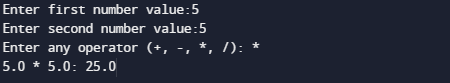In the above program, we have first declared and initialized a set variables required in the program.

• a= it will hold First value.
• b= it will hold second value.
• op=it will hold operator value.

And in the next statement user will be prompted to enter two values and  which will be assigned to variable ‘a‘ and ‘b‘ respectively.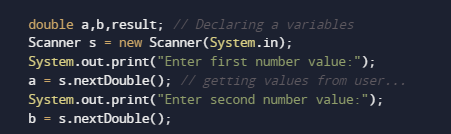And after taking the values of a and b we will ask user to input symbol to perform calculation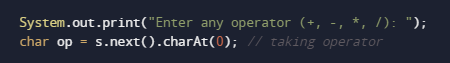After taking all the  values and sign in op we will pass the value of op to switch case condition Calculate values of a operation pass to switch case.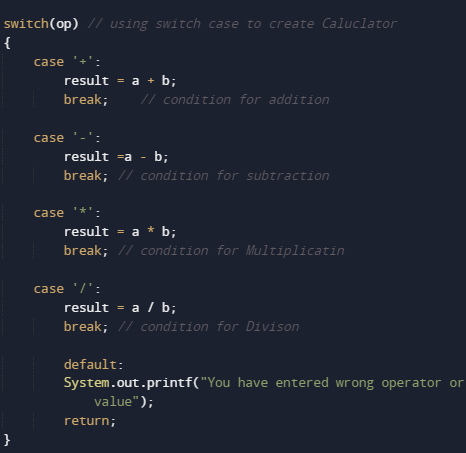The above switch case compute the Multiplication of two numbers and print the output.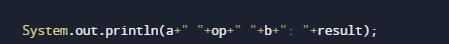And  with the break statement  compiler ends the switch statement and operation given different different operators can be performed.

In this tutorial we have learn about the Java Program to make a calculator using switch case and its application with practical example. I hope you will like this tutorial.